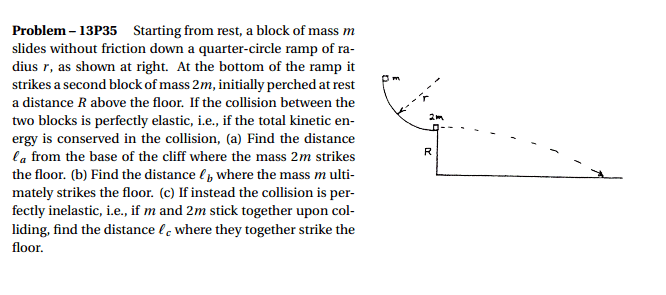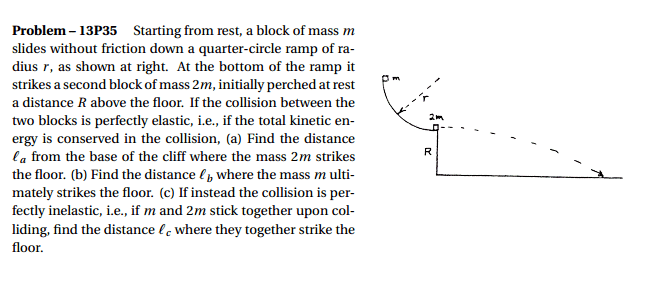# Inelastic collisions - conservation of energy

## Homework StatementK = E + U
E = 1/2mv^2
U = mgh

## The Attempt at a Solution

So I think that for a, half the final velocity of m is equal to the initial velocity of 2m. So that's v = sqrt(2gr)/2? Momentum is not conserved because normal force and gravity are acting on block m correct? Then what do I do from there, and on b and c?

SammyS
Staff Emeritus
Science Advisor
Homework Helper
Gold Member

## Homework StatementK = E + U
E = 1/2mv^2
U = mgh

## The Attempt at a Solution

So I think that for a, half the final velocity of m is equal to the initial velocity of 2m. So that's v = sqrt(2gr)/2? Momentum is not conserved because normal force and gravity are acting on block m correct? Then what do I do from there, and on b and c?

This is a multi-stage problem.

Attack it systematically.

First, the block with mass, m, slides down the ramp. What is its velocity immediately before it collides with the block of mass 2m ?

Then there is the collision.

What velocity does each block have immediately after the collision?

The rest of this depends upon those velocities.

So then,
mgr = 1/2mv1 + 1/2(2m)v2 right?
So, v2 = sqrt(gr-1/2(v1)^2)

From the kinematics formula, the time it takes for the block to fall is
R = 1/2gt^2
So t = sqrt(2R/g)

But my book says the answer is 4/3*sqrt(Rr), but v2*t is clearly not the same...what am I doing wrong?

SammyS
Staff Emeritus
Science Advisor
Homework Helper
Gold Member
So then,
mgr = 1/2mv1 + 1/2(2m)v2 right?
So, v2 = sqrt(gr-1/2(v1)^2)

From the kinematics formula, the time it takes for the block to fall is
R = 1/2gt^2
So t = sqrt(2R/g)

But my book says the answer is 4/3*sqrt(Rr), but v2*t is clearly not the same...what am I doing wrong?
Which answer?

It appears that you have not taken care to go though each step that's involved here.

You do have the speed of the small mass immediately prior to the collision correct.

Then there is the collision.

How do you propose to analyze that? It's an elastic collision. What velocity does each mass have immediately after the collision (including direction).
Hint: Yes, you need to use conservation of momentum.​

After that, you might begin to concern yourself with the distances asked for.

After that you